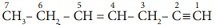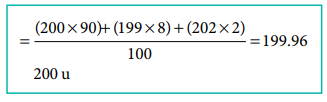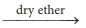# TN 11TH CHEMISTRY - Online Test

Q1. Gases deviate from ideal behavior at high pressure. Which of the following statement(s) is correct for non-ideality?
Explaination / Solution:
No Explaination.

Q2. If Kb and Kf for a reversible reactions are 0.8 ×10–5 and 1.6 × 10–4 respectively, the value of the equilibrium constant is,
Explaination / Solution:

Kb = 0.8 × 10–5

Kf = 1.6 × 10–4

Keq = Kf / Kb = 1.6X10-4 / 0.8X10-5 = 20

Q3. The amount of heat exchanged with the surrounding at constant temperature and pressure is given by the quantity
Explaination / Solution:
No Explaination.

Q4. The molality of a solution containing 1.8g of glucose dissolved in 250g of water is
Explaination / Solution:

molality = number of moles of solute / weight of solvent (in kg)

= (1.8/180) / 0.25 = 0.01/0.25 = 0.04M

Q5. In which of the following Compounds does the central atom obey the octet rule?
Explaination / Solution:

Compound  : No. of valance electron on the central atom

XeF2 : 10

AlCl3 : 6

SF6 : 12

SCl2 : 8

Q6.

In the hydrocarbonstate of hybridisation of carbon 1,2,3,4 and 7 are in the following sequence.

Explaination / Solution:
No Explaination.

Q7. What is the hybridisation state of benzyl­ carbonium ion?
Explaination / Solution:
No Explaination.

Q8. An element X has the following isotopic composition 200X = 90 %, 199X = 8 % and 202X = 2 %. The weighted average atomic mass of the element X is closest to
Explaination / Solution:Q9. C2H5 Br + 2Na→ C4H10 + 2NaBr

The above reaction is an example of which of the following

Explaination / Solution:
No Explaination.

Q10. The energy of light of wavelength 45 nm is
Explaination / Solution:

E = hν = hc/λ

=6.626 × 1034 J s × 3 ×108 m s1  / 45 ×109 m

= 4 .42 ×1018 J